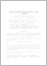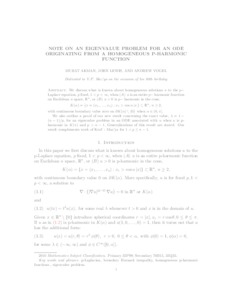# Note on an Eigenvalue problem for an ODE originating from a homogeneous p-harmonic function

Akman, Murat and Lewis, John and Vogel, Andrew (2019) 'Note on an Eigenvalue problem for an ODE originating from a homogeneous p-harmonic function.' Algebra i Analiz, 31 (2). pp. 75-87. ISSN 0234-0852Preview
Text
ALV Eigenvalue.pdf - Submitted Version

We discuss what is known about homogeneous solutions $u$ to the p-Laplace equation, $p$ fixed, $1 < p < \infty,$ when $u$ is an entire p-harmonic function in $\mathbb{R}^{n}$, </ul> or $u > 0$ is p-harmonic in the cone, $K(\alpha) = \{ x = (x_1, \dots, x_n ) : x_1 > \cos \alpha \, | x| \} \subset \mathbb{R}^n, \, n \geq 2,$ with continuous boundary value zero on $\partial K (\alpha) \setminus \{0\}$ when $\alpha \in (0, \pi].$ We also outline a proof of our new result concerning the exact value, $\lambda = 1 - (n-1)/p,$ for an eigenvalue problem in an ODE associated with $u$ when $u$ is p-hamonic in $K ( \pi )$ and $p > n - 1.$ Generalizations of this result are stated for $\lambda \leq n - 1.$ Our result complements work of Krol'-Maz'ya in [KM] for $1 < p \leq n - 1.$View Item